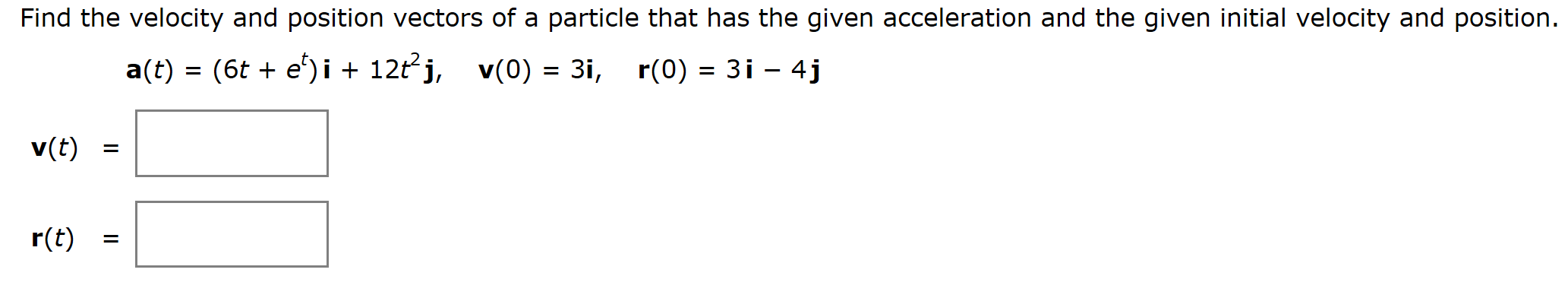# Find the velocity and position vectors of a particle that has the given acceleration and the given initial velocity and positiona(t) (6t e')i + 12t j, v(0) = 3i, r(0) 3i - 4jv(t)r(t)

Question
3 viewshelp_outlineImage TranscriptioncloseFind the velocity and position vectors of a particle that has the given acceleration and the given initial velocity and position a(t) (6t e')i + 12t j, v(0) = 3i, r(0) 3i - 4j v(t) r(t) fullscreen
check_circle

Step 1

To calculate the velocity and position function for the particle.

Step 2

Given:

Step 3

Formula Used:

...

### Want to see the full answer?

See Solution

#### Want to see this answer and more?

Solutions are written by subject experts who are available 24/7. Questions are typically answered within 1 hour.*

See Solution
*Response times may vary by subject and question.
Tagged in

### Functions